Search

About 26 Search Results Matching Types of Worksheet, Worksheet Section, Generator, Generator Section, Subjects matching Math, Type matching Worksheet Section, Grades matching 3rd Grade, Similar to Easy Measurement Worksheets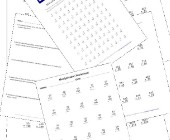Multiplication Worksheets for Kids

This set of math worksheets is geared towards help...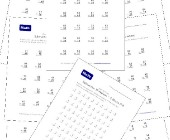Subtraction Worksheets for Kids

This set of free math worksheets is geared towards...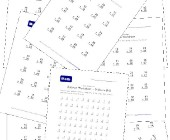This collection of addition worksheets includes si...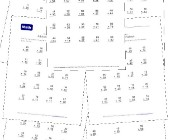Math Help - Addition Worksheets

This group of addition worksheets included a varie...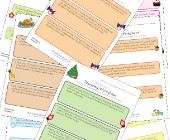Rounding Worksheets

Check out our collection of rounding worksheets in...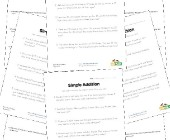Word Problem Worksheets

Help children develop their problem solving skills...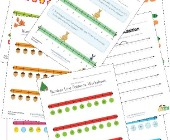Number Line Worksheets

Check out our collection of number line worksheets...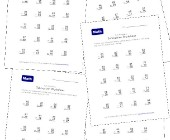Subtraction Worksheets - With Borrowing

This set of subtraction worksheets focuses on help...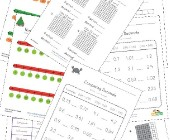Decimal Worksheets

This collection of free decimal worksheets will he...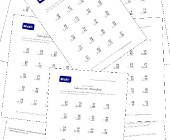Math Worksheets - Two Digit Subtraction

This group of subtraction worksheets is another gr...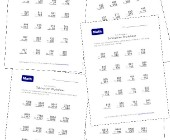Math Help Worksheets - Practice Subtraction

This group of subtraction worksheets includes a va...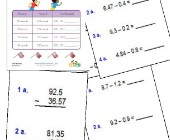Subtracting Decimals Worksheets

These worksheets will help children practice decim...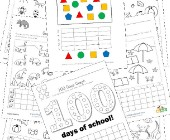Graphing Worksheets

Take a look at our collection of graphing workshee...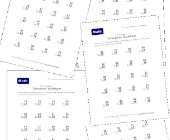Subtraction Worksheets - Without Borrowing

Check out this set of subtraction worksheets if yo...